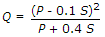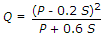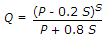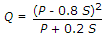# Civil Engineering - Water Resources Engineering

### Exercise :: Water Resources Engineering - Section 1

31.

The run off a drainage basin is

 A. Initial recharge + ground water accretion + precipitation B. Precipitation + ground water accretion + initial recharge C. Precipitation - ground water accretion + initial recharge D. Precipitation - ground water accretion - initial recharge.

Explanation:

No answer description available for this question. Let us discuss.

32.

For computing the run off volumes of large areas, number of infiltrations used are

 A. 2 B. 3 C. 4 D. 5

Explanation:

No answer description available for this question. Let us discuss.

33.

From the Survey of India map, the distance of the critical point is 20 km and difference in elevation is 193 m. The over land flow time, is

 A. 2 hours B. 3 hours C. 2 hours and 30 minutes D. 3 hours and 30 minutes E. 4 hours.

Explanation:

No answer description available for this question. Let us discuss.

34.

If the area of storm hydrograph is equal to 102 cm, the ordinates of a unit hydrograph may be obtained by dividing the ordinates of the storm hydrograph by

 A. 0.5 B. 1 C. 2 D. 3 E. 4

Explanation:

No answer description available for this question. Let us discuss.

35.

If s is the potential infiltration, P is rainfall in cm in a drainage of a soil with fair pasture cover, the direct run off Q in cm is given by

 A.B.C.D.